Processing ......FreeComputerBooks.com Links to Free Computer, Mathematics, Technical Books all over the World

An Introduction to Combinatorics and Graph Theory
• Title: An Introduction to Combinatorics and Graph Theory
• Authors David Guichard
• Publisher: David Guichard (February 18, 2017); eBook (Creative Commons Licensed)
• Paperback: N/A
• eBook: HTML and PDF
• Language: English
• ISBN-10: N/A
• ISBN-13: N/A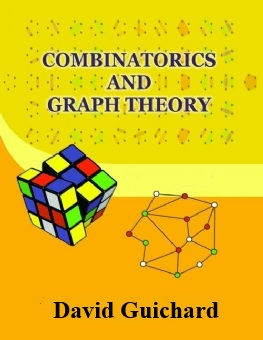Book Description

Combinatorics is a branch of mathematics concerning the study of finite or countable discrete structures. Aspects of combinatorics include counting the structures of a given kind and size (enumerative combinatorics), deciding when certain criteria can be met, and constructing and analyzing objects meeting the criteria (as in combinatorial designs and matroid theory), finding "largest", "smallest", or "optimal" objects (extremal combinatorics and combinatorial optimization), and studying combinatorial structures arising in an algebraic context, or applying algebraic techniques to combinatorial problems (algebraic combinatorics).

Graph theory is the study of graphs, which are mathematical structures used to model pairwise relations between objects. A "graph" in this context is made up of "vertices" or "nodes" and lines called edges that connect them. A graph may be undirected, meaning that there is no distinction between the two vertices associated with each edge, or its edges may be directed from one vertex to another; see graph (mathematics) for more detailed definitions and for other variations in the types of graph that are commonly considered. Graphs are one of the prime objects of study in discrete mathematics.

This book walks the reader through the classic parts of Combinatorics and graph theory, while also discussing some recent progress in the area: on the one hand, providing material that will help students learn the basic techniques, and on the other hand, showing that some questions at the forefront of research are comprehensible and accessible to the talented and hardworking undergraduate.

• David Guichard is a professor of mathematics in the department of Mathematics and Computer Science at Whitman College. His research interests are combinatorics and graph theory.
Reviews, Ratings, and Recommendations: Related Book Categories: Read and Download Links:Similar Books:
•Combinatorics Through Guided Discovery (Kenneth P. Bogart)

This book is an introduction to combinatorial mathematics, also known as combinatorics. The book focuses especially but not exclusively on the part of combinatorics that mathematicians refer to as 'counting'.

•Applied Combinatorics (Mitchel T. Keller, et al)

This is a text with more than enough material for a one-semester introduction to combinatorics. The original target audience was primarily computer science majors, but the topics included make it suitable for a variety of different students.

•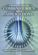Foundations of Combinatorics with Applications (Edward Bender)

This introduction to combinatorics, the foundation of the interaction between computer science and mathematics, is suitable for upper-level undergraduates and graduate students in engineering, science, and mathematics.

•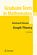Graph Theory (Reinhard Diestel)

This book covers the core material of the subject with concise yet reliably complete proofs, while offering glimpses of more advanced methods in each field by one or two deeper results, again with proofs given in full detail.

•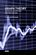Graph Theory - Advanced Algorithms and Applications

Not only will the methods and explanations help you to understand more about graph theory, but you will find it joyful to discover ways that you can apply graph theory in your applications or scientific research.

•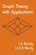Graph Theory with Applications (J.A. Bondy, et al.)

The primary aim of this book is to present a coherent introduction to graph theory, suitable as a textbook for advanced undergraduate and beginning graduate students in mathematics and computer science.

•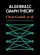Explorations in Algebraic Graph Theory (Chris Godsil, et al.)

This book aims to express properties of graphs in algebraic terms, then to deduce theorems about them. It tackles the applications of linear algebra and matrix theory to the study of graphs; algebraic constructions such as adjacency matrix, using the Sage.

•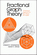Fractional Graph Theory: A Rational Approach (E. Scheinerman)

In this book the authors explore generalizations of core graph theory notions by allowing real values to substitute where normally only integers would be permitted. The aim is to prove "fractional analogues" of the theorems of traditional graph theory.

Book Categories
 :All CategoriesRecent BooksMiscellaneous BooksComputer LanguagesComputer ScienceData Science/DatabasesElectrical EngineeringJava and Java EE (J2EE)Linux and UnixMathematicsMicrosoft and .NETMobile ComputingNetworking and CommunicationsSoftware EngineeringSpecial TopicsWeb Programming
Other Categories